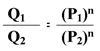Calculators

Flow Rate Calculator

Use this calculator to determine the flow rate of any nozzle at any operating pressure. Inputs to this calculator are the nozzle type, current operating pressure and flow, desired flow or desired pressure.

Equation

Nozzle flow rate varies with spraying pressure. In general, the relationship between flow rate and pressure is as follows:* Required field

Not sure?

Not sure? Specific gravity is the ratio of the density of a fluid compared to the density of water. The specific gravity of water is 1. For more information on how to calculate specific gravity, contact your local sales person.

The spray pressure required to achieve the desired flow rate is

The flow rate at the given pressure is

GPM L/MIN

* Required field